# Long-Term EU GDP Growth Rate by Region, Second Quarter 2021

Jan 3, 2022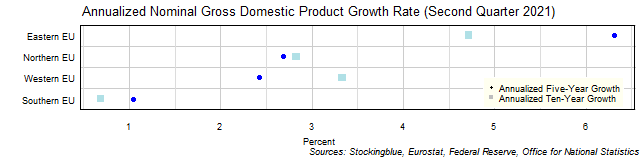The chart above shows the annualized nominal gross domestic product (GDP) growth rate in each EU region over the past five years as of the second quarter of 2021 and the growth rate over the past ten years.  All four regions grew over both time periods.

# Long-Term US GDP Growth Rate by Region, Second Quarter 2021

Dec 31, 2021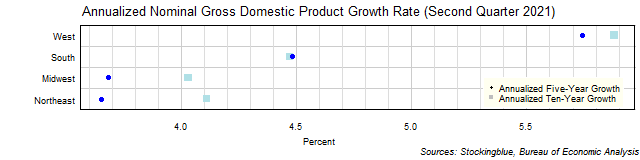The chart above shows the annualized nominal gross domestic product (GDP) growth rate in each US region over the past five years as of the second quarter of 2021 and the growth rate over the past ten years.  All four regions grew over both time periods.

# Long-Term EU and US GDP Growth Rate by State, Second Quarter 2021

Dec 30, 2021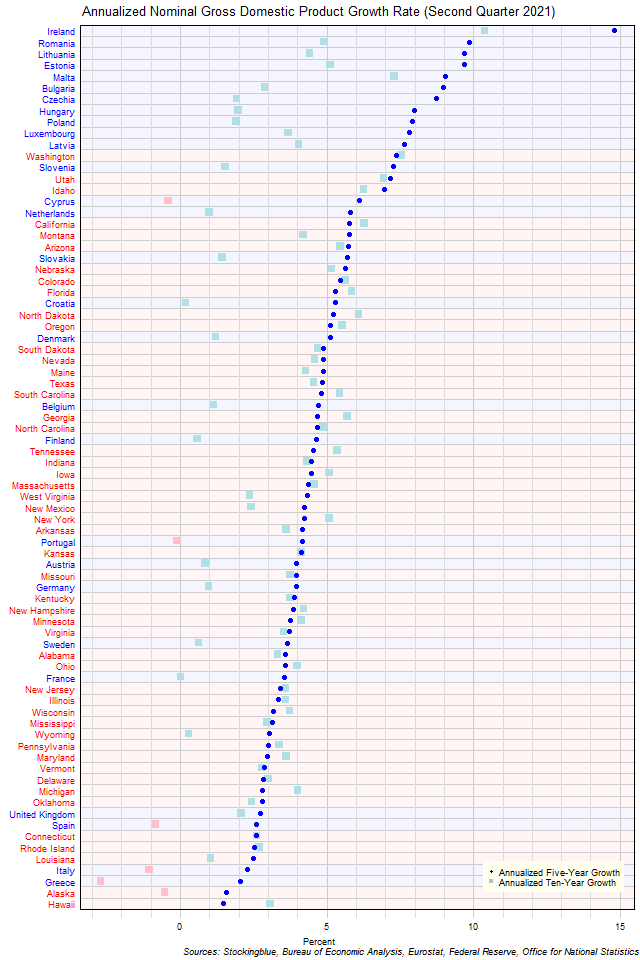The chart above shows the annualized nominal gross domestic product (GDP) growth rate when the GDP is priced in US dollars in each EU and US state over the past five years as of the second quarter of 2021 and the growth rate over the past ten years.  Six states had negative growth over the past ten years while zero had negative growth over the past five.

# Long-Term EU GDP Growth Rate by State, Second Quarter 2021

Dec 29, 2021The chart above shows the annualized nominal gross domestic product (GDP) growth rate in each EU state over the past five years as of the second quarter of 2021 and the growth rate over the past ten years.  One state experienced negative growth either in the past five years or in the past ten years.

# Long-Term US GDP Growth Rate by State, Second Quarter 2021

Dec 28, 2021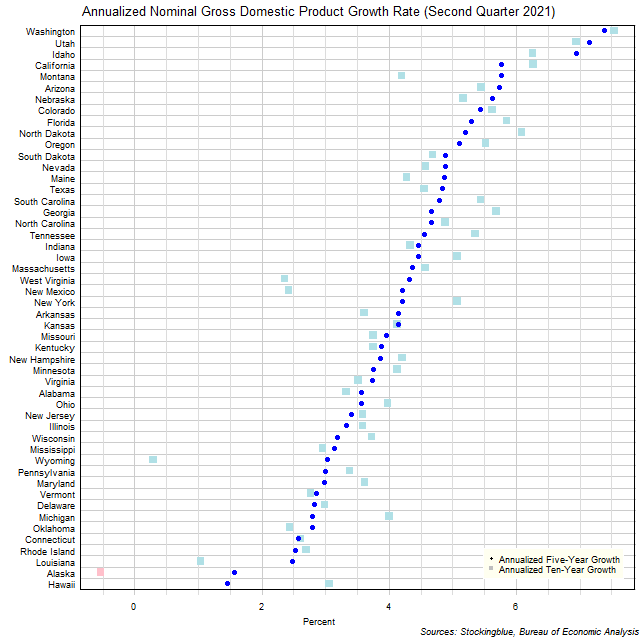The chart above shows the annualized nominal gross domestic product (GDP) growth rate in each US state over the past five years as of the second quarter of 2021 and the growth rate over the past ten years.  All states experienced growth over the past five years.

# Long-Term Per Capita GDP by EU and US Region, Second Quarter 2021

Dec 27, 2021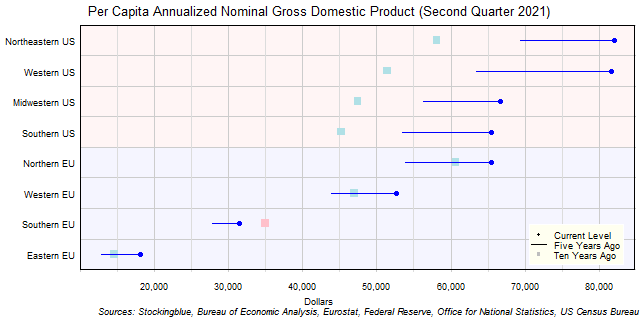The chart above shows the per capita annualized nominal gross domestic product (GDP) in each EU and US region as of the second quarter of 2021 in US dollars, the change from five years ago, and the per capita GDP ten years prior.  Only one EU region has seen a drop in per capita GDP over the past five or ten years.

# Long-Term Per Capita GDP by EU Region, Second Quarter 2021

Dec 24, 2021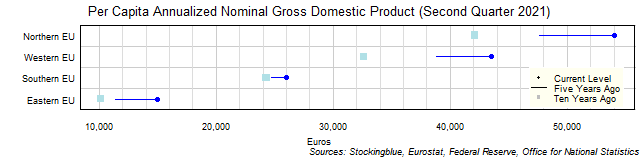The chart above shows the per capita annualized nominal gross domestic product (GDP) in each EU region as of the second quarter of 2021 in euros, the change from five years ago, and the per capita GDP ten years prior.  Every single region's economy grew both over the past five and past ten years.

# Long-Term Per Capita GDP by US Region, Second Quarter 2021

Dec 23, 2021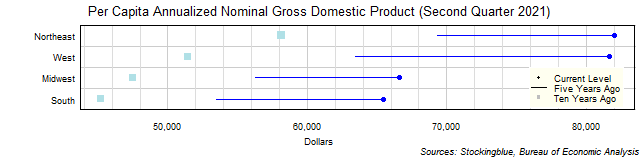The chart above shows the per capita annualized nominal gross domestic product (GDP) in each US region as of the second quarter of 2021 in dollars, the change from five years ago, and the per capita GDP ten years prior.  Every single region's economy grew both over the past five and past ten years.

# Long-Term Per Capita GDP by EU and US State, Second Quarter 2021

Dec 22, 2021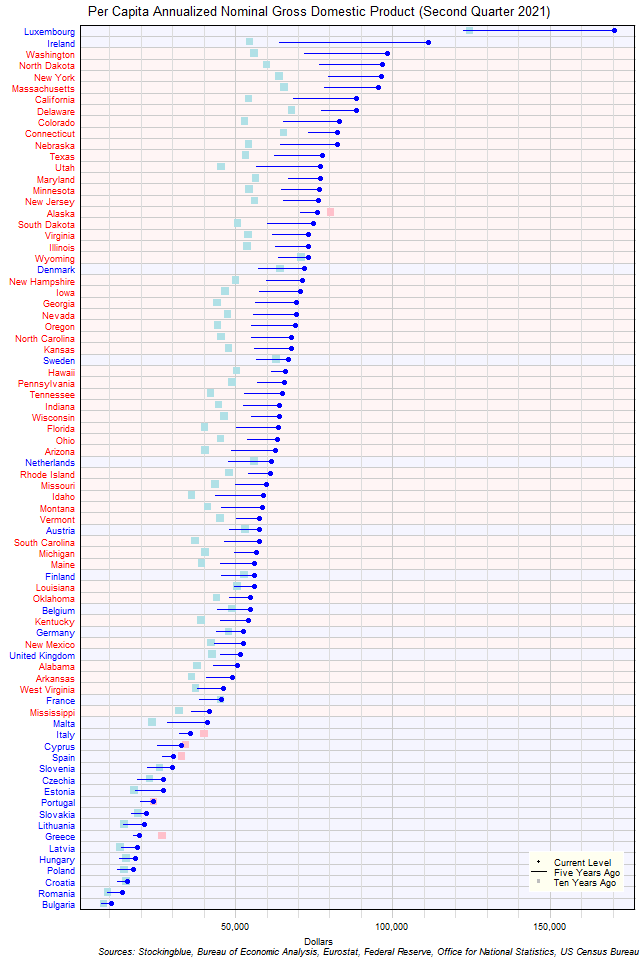The chart above shows the per capita annualized nominal gross domestic product (GDP) in each EU and US state as of the second quarter of 2021 in dollars, the change from five years ago, and the GDP ten years prior.  There are six states that have a lower per capita GDP now than ten years ago.

# Long-Term Per Capita GDP by EU State, Second Quarter 2021

Dec 21, 2021The chart above shows the per capita annualized nominal gross domestic product (GDP) in each EU state as of the second quarter of 2021 in euros, the change from five years ago, and the per capita GDP ten years prior.  Luxembourg and Ireland had exceptional growth over the past five years.